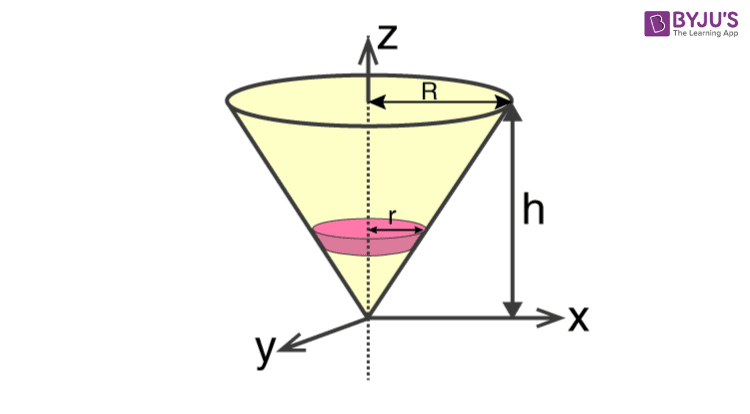Checkout JEE MAINS 2022 Question Paper Analysis : Checkout JEE MAINS 2022 Question Paper Analysis :

# Moment Of Inertia Of Solid Cone

Moment of inertia of solid cone can be expressed using the given formula;

 I = 3 MR2/ 10

However, in this lesson, we will understand how the formula is derived and used in solving the problems. Let us first go through the derivation of the moment of inertia formula for a solid cone.

## Solid Cone Moment Of Inertia Formula Derivation

We will take a solid cone where its axis will pass through the centre with radius = r, height = h. We will divide the cone into a small elemental disc where we consider the cone’s radius to be r and of thickness dz. We will need to determine the mass though.We take the elemental disc whose mass is given by;

dm = ρ ⋅ π r2dz

Density is given as;

ρ = M / V = M / (⅓ π R2h)

With this, we will calculate the dm.

dm = [M / (⅓ π R2h)] . ( π r2dz)

dm = (3M / R2h) . (r2dz)

If we consider the similarity of the triangle, then we have;

R / r = h / z

r = R X (z / h)

Now,

dm = (3M / R2h ) . (R2 ⋅ z2/ h2 )dz

dm = (3M z2 / h3) dz

If we consider z-axis, the moment of inertia of the elemental disk will be;

dI = (½) dmr2

dI = (½ )⋅ (3M z2/ h3 ) ⋅ (z2 R2 / h2 )dz

dI = (3 M R2 /2 h5 ).  zdz

Now we will follow the integration process. Here;

I =(3 M R2 /2 h5 )  oh z4dz

I = (3 M R2 /2 h5 ). [z5 / 5 ]oh

I = (3 M R2 /2 h5 )⋅ (h5 / 5)

Therefore, I = 3 MR2/10